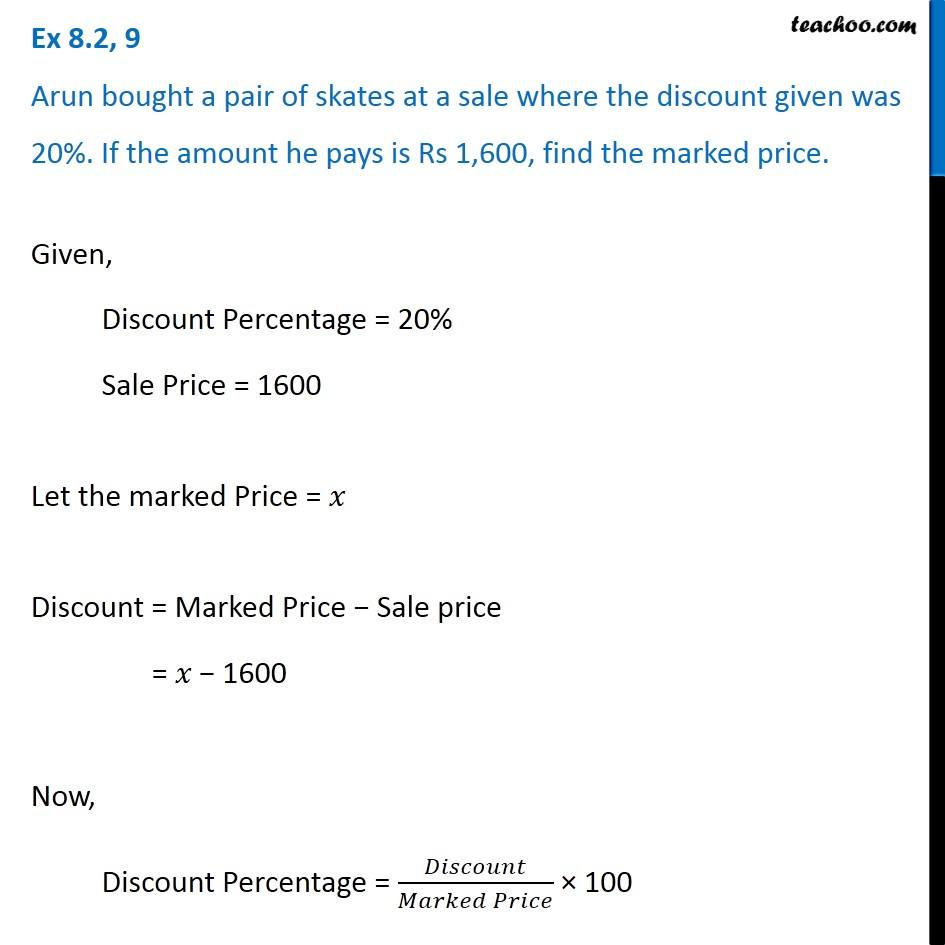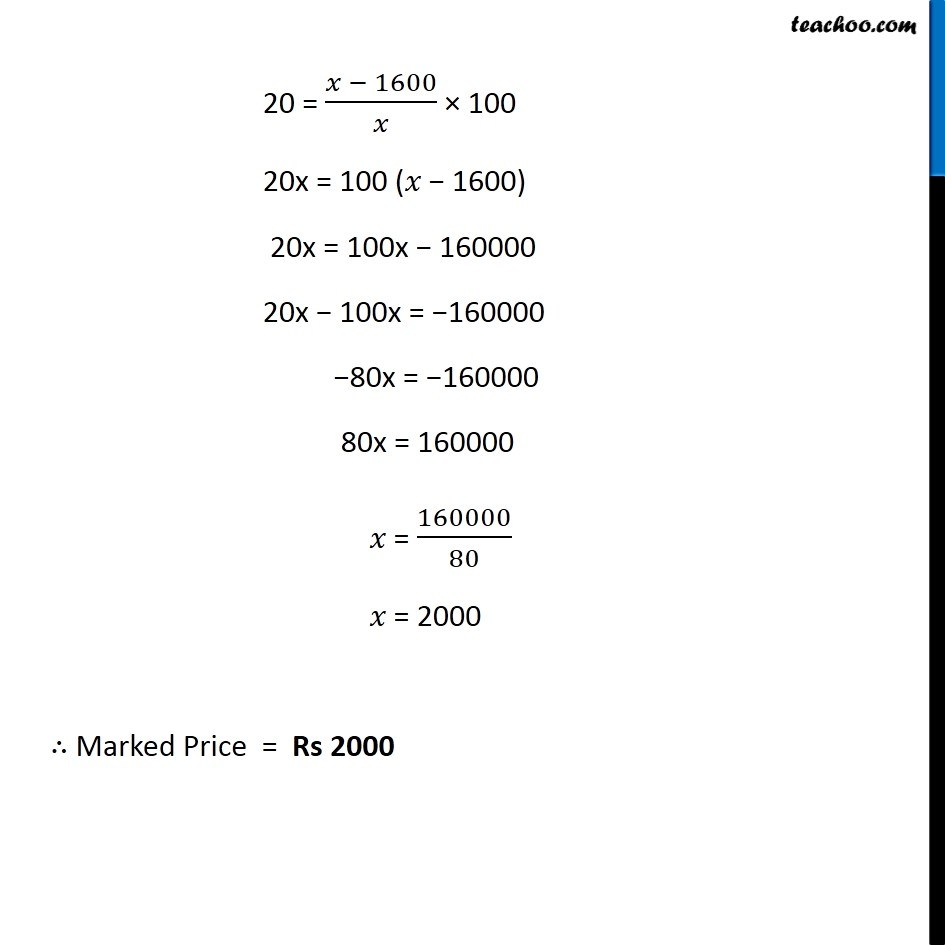1. Chapter 8 Class 8 Comparing Quantities
2. Concept wise
3. Finding Discounts

Transcript

Ex 8.2, 9 Arun bought a pair of skates at a sale where the discount given was 20%. If the amount he pays is Rs 1,600, find the marked price. Given, Discount Percentage = 20% Sale Price = 1600 Let the marked Price = 𝑥 Discount = Marked Price − Sale price = 𝑥 − 1600 Now, Discount Percentage = 𝐷𝑖𝑠𝑐𝑜𝑢𝑛𝑡/(𝑀𝑎𝑟𝑘𝑒𝑑 𝑃𝑟𝑖𝑐𝑒) × 100 20 = (𝑥 − 1600)/𝑥 × 100 20x = 100 (𝑥 − 1600) 20x = 100x − 160000 20x − 100x = −160000 −80x = −160000 80x = 160000 𝑥 = 160000/80 𝑥 = 2000 ∴ Marked Price = Rs 2000

Finding Discounts